# ML Aggarwal Class 8 Solutions for ICSE Maths Chapter 4 Cubes and Cube Roots Ex 4.1

## ML Aggarwal Class 8 Solutions for ICSE Maths Chapter 4 Cubes and Cube Roots Ex 4.1

Question 1.
Which of the following numbers are not perfect cubes? Give reasons in support of your answer:
(i) 648
(ii) 729
(iii) 8640
(iv) 8000
Solution: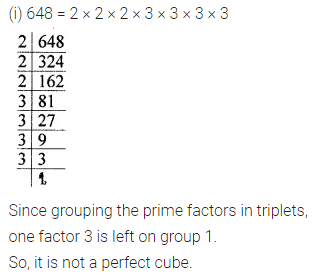Question 2.
Show that each of the following numbers is a perfect cube. Also, find the number whose cube is the given number:
(i) 1728
(ii) 5832
(iii) 13824
(iv) 35937
Solution: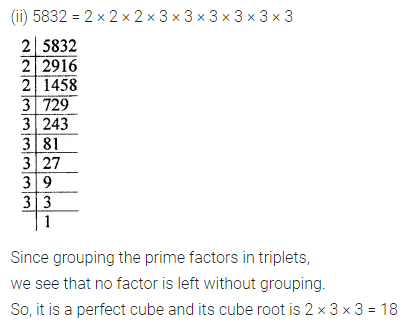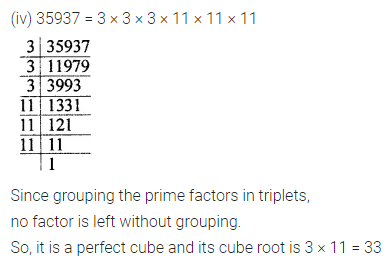Question 3.
Find the smallest number by which each of the following numbers must be multiplied to obtain a perfect cube:
(i) 243
(ii) 3072
(iii) 11979
(iv) 19652
Solution: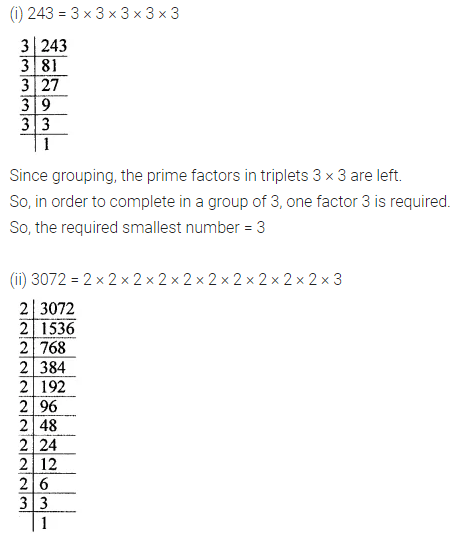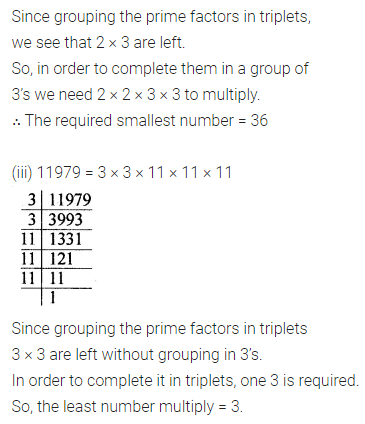Question 4.
Find the smallest number by which each of the following numbers must be divided to obtain a perfect cube:
(i) 1536
(ii) 10985
(iii) 28672
(iv) 13718
Solution: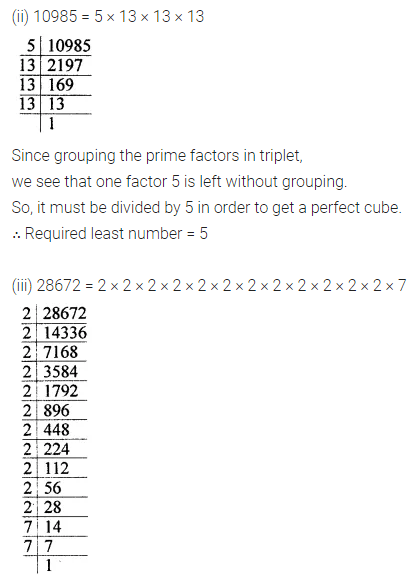Question 5.
Rahul makes a cuboid of plasticine of sides 3 cm × 3 cm × 5 cm. How many such cuboids will he need to form a cube?
Solution:Question 6.
Find the volume of a cubical box whose surface area is 486 cm2.
Solution:Question 7.
Which of the following are cubes of even natural numbers or odd natural numbers:
(i) 125
(ii) 512
(iii) 1000
(iv) 2197
(v) 4096
(vi) 6859
Solution:Question 8.
Write the ones digit of the cube of each of the following numbers:
(i) 231
(ii) 358
(iii) 419
(iv) 725
(v) 854
(vi) 987
(vii) 752
(viii) 893
Solution: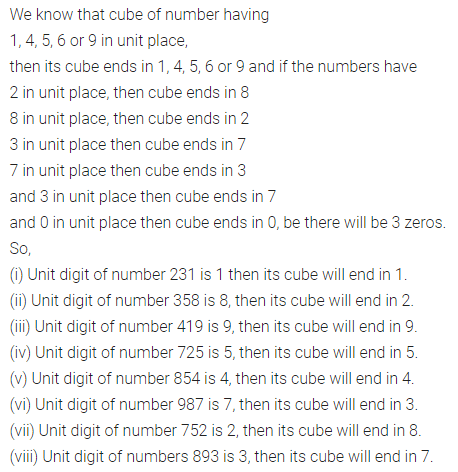Question 9.
Find the cubes of the following numbers:
(i) 13
(ii) $$3 \frac{1}{5}$$
(iii) $$-5 \frac{1}{7}$$
Solution: